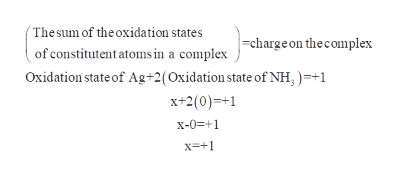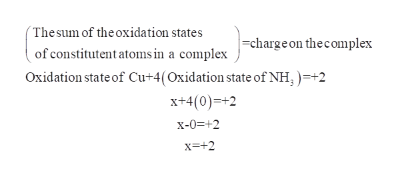# Identify each of the following coordination complexes as either diamagnetic or paramagnetic.[Ag(NH3)2]+Square planar [Cu(NH3)4]2+[Ru(bipy)3]2+[CoCl4]2-

Question
1 views
• Identify each of the following coordination complexes as either diamagnetic or paramagnetic.
1. [Ag(NH3)2]+
2. Square planar [Cu(NH3)4]2+
3. [Ru(bipy)3]2+
4. [CoCl4]2-
check_circle

Step 1

To determine magnetic behavior of complexes:

The following coordination complexes are

a). (Ag (NH3)2)+

The atomic number of silver is 47 so, electronic configuration of silver is (Kr) 4d105s1.

The oxidation state of ammonia molecule is 0.

Assume oxidation state of silver as x.

By calculating the oxidation state of silver in the given formula, we get as +1.help_outlineImage Transcriptionclose(Thesum of the oxidation states =charge on thecomplex of constitutent atoms in a complex Oxidation state of Ag+2(Oxidation state of NH, )=+1 x+2(0)=+1 x-0=+1 x=+1 fullscreen
Step 2
• In +1 oxidation state its electronic configuration will be (Kr) 4d105s0.
• The outermost electronic arrangements of electrons are given.
• Silver metal shares a pair of electrons from each ammonia molecule. Hence, the two electron pairs donated by the ligand go to the outermost orbitals as shown.

Therefore, the complex (Ag (NH3)2)+ has no unpaired electrons and so it is diamagnetic.

Step 3

b). Square planar (Cu (NH3)4)2+

The atomic number of copper is 29 so, electronic configuration of copper is (Ar) 3d104s1.

The oxidation state of ammonia is 0.

Assume oxidation ...help_outlineImage Transcriptionclose(Thesum of the oxidation states =charge on thecomplex of constitutent atoms in a complex Oxidation state of Cu+4(Oxidation state of NH; )=+2 x+4(0)=+2 x-0=+2 X=+2 fullscreen

### Want to see the full answer?

See Solution

#### Want to see this answer and more?

Solutions are written by subject experts who are available 24/7. Questions are typically answered within 1 hour.*

See Solution
*Response times may vary by subject and question.
Tagged in

### Inorganic Chemistry